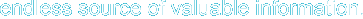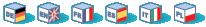# Introduction

These functions allow you to work with arbitrary-length integers using the GNU MP library.

These functions have been added in PHP 4.0.4.

Note: Most GMP functions accept GMP number arguments, defined as resource below. However, most of these functions will also accept numeric and string arguments, given that it is possible to convert the latter to a number. Also, if there is a faster function that can operate on integer arguments, it would be used instead of the slower function when the supplied arguments are integers. This is done transparently, so the bottom line is that you can use integers in every function that expects GMP number. See also the gmp_init() function.

 Warning If you want to explicitly specify a large integer, specify it as a string. If you don't do that, PHP will interpret the integer-literal first, possibly resulting in loss of precision, even before GMP comes into play.

Note: This extension is available on Windows platforms since PHP 5.1.0.

# Requirements

You can download the GMP library from http://www.swox.com/gmp/. This site also has the GMP manual available.

You will need GMP version 2 or better to use these functions. Some functions may require more recent version of the GMP library.

# Installation

In order to have these functions available, you must compile PHP with GMP support by using the --with-gmp option.

# Runtime Configuration

This extension has no configuration directives defined in php.ini.

# Resource Types

This extension has no resource types defined.

# Predefined Constants

The constants below are defined by this extension, and will only be available when the extension has either been compiled into PHP or dynamically loaded at runtime.

GMP_ROUND_ZERO (integer)

GMP_ROUND_PLUSINF (integer)

GMP_ROUND_MINUSINF (integer)

# Examples

Example 1. Factorial function using GMP

 ``` ```

This will calculate factorial of 1000 (pretty big number) very fast.

More mathematical functions can be found in the sections BCMath Arbitrary Precision Mathematics Functions and Mathematical Functions.

gmp_abs -- Absolute value
gmp_and -- Logical AND
gmp_clrbit -- Clear bit
gmp_cmp -- Compare numbers
gmp_com -- Calculates one's complement
gmp_div_q -- Divide numbers
gmp_div_qr -- Divide numbers and get quotient and remainder
gmp_div_r -- Remainder of the division of numbers
gmp_div -- Alias of gmp_div_q()
gmp_divexact -- Exact division of numbers
gmp_fact -- Factorial
gmp_gcd -- Calculate GCD
gmp_gcdext -- Calculate GCD and multipliers
gmp_hamdist -- Hamming distance
gmp_init -- Create GMP number
gmp_intval -- Convert GMP number to integer
gmp_invert -- Inverse by modulo
gmp_jacobi -- Jacobi symbol
gmp_legendre -- Legendre symbol
gmp_mod -- Modulo operation
gmp_mul -- Multiply numbers
gmp_neg -- Negate number
gmp_or -- Logical OR
gmp_perfect_square -- Perfect square check
gmp_popcount -- Population count
gmp_pow -- Raise number into power
gmp_powm -- Raise number into power with modulo
gmp_prob_prime -- Check if number is "probably prime"
gmp_random -- Random number
gmp_scan0 -- Scan for 0
gmp_scan1 -- Scan for 1
gmp_setbit -- Set bit
gmp_sign -- Sign of number
gmp_sqrt -- Calculate square root
gmp_sqrtrem --  Square root with remainder
gmp_strval -- Convert GMP number to string
gmp_sub -- Subtract numbers
gmp_xor -- Logical XOR

Hosting by: Hurra Communications Ltd.
Generated: 2007-01-26 18:00:28